# Wideband 180 Degree Coupler

Click here to go to our main page on couplers and splitters

Click here to go to our main page on hybrid 3dB couplers, including 180 degree designs

New for February 2019: This page describes a unique 180-degree coupler based on asymmetric coupled lines, by loyal MW101-er Carl Pfeiffer, who has written extensively in peer-reviewed journals. He says:

I had to design a wideband 180o coupler and I was not able to find any information on the Microwaves 101 website. Since the 180 coupler is a fundamental component in microwave engineering I figured it would be useful to have some design information on the Microwaves 101 website.

Thanks, Carl!

The rat race coupler is a simple method of realizing a 180 degree hybrid coupler. However, it has a narrow bandwidth on the order of 20%. One method of realizing a wideband 180 degree hybrid is to use asymmetric coupled transmission lines. These couplers commonly realize 10:1 bandwidth ratios. The disadvantage of this approach is they are large and generally require a multilayer PCB for fabrication.

The theory behind this coupler can be found in Pozar’s Microwave Engineering, Chapter 7: Power dividers and directional couplers (among other places). As pointed out in the coupled line couplers section (https://www.microwaves101.com/encyclopedias/coupled-line-couplers), impedance matched, symmetric, lossless structures have a 90 degree phase difference between the output ports. Therefore, 180 degree couplers use asymmetric coupled transmission lines. The typical implementation is shown below using offset stripline configuration (drawing not to scale). The tapered lines are coupled with an even mode impedance equal to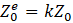and the odd mode impedance equal to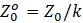. At the left, the lines are very weakly coupled (i.e., k=1), and the coupling gradually increases towards the right. Ideally, the lines are adiabatically coupled such that there are no reflections from the increasing coupling coefficient of the lines leading up to the junction. The Klopfenstein taper is typically used here to provide a wideband impedance match. At the right, there is a junction between strongly coupled lines and the uncoupled lines leading to ports 4 and 2. The transmission lines leading to ports 4 and 2 are important for proper 0 degree and 180 degree phasing at the output ports (2 and 3). For 3 dB couplers, the peak coupling coefficient of the lines is k=5.8 at the junction. Note that there is a strong reflection at the junction, which is of fundamental importance to how the coupler works. I could go into the math behind the even and odd mode excitations and how they lead to 180 degree and 0 degree phase difference at the output ports (2 and 3) when ports 1 and 4 are fed, but I will leave that to the many textbooks on the subject (e.g. Pozar).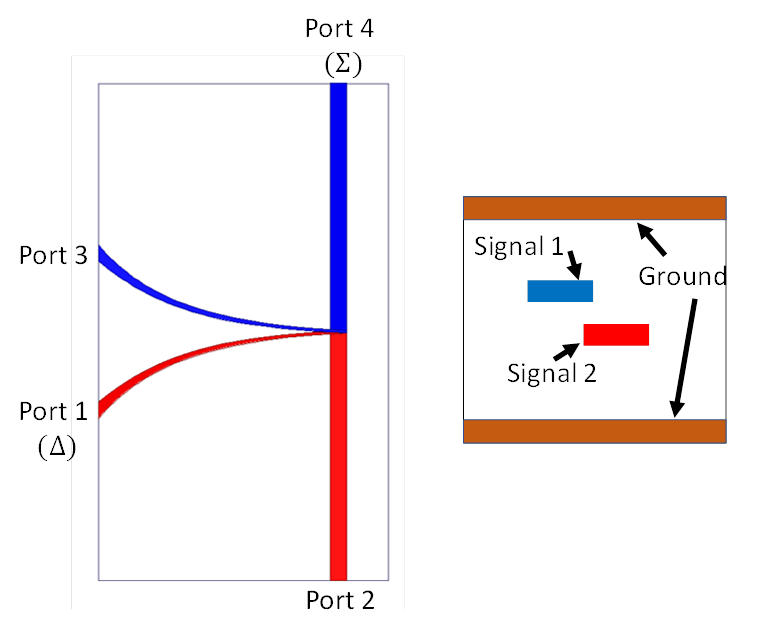A circuit model of a 3 dB coupled line 180 degree hybrid is shown below. This schematic was made in Qucs (Quite Universal Circuit Simulator), which is an open source circuit simulator I like to use when I am at home. The uncoupled lines on the left are impedance matched to strongly coupled lines leading up to the junction on the right. The max coupling coefficient is k=5.8. The impedances along the lines were calculated using the Klopfenstein taper calculator on the Microwaves 101 download section.  The length of the Klopfenstein taper was chosen to have a 30 dB return loss above 2 GHz. The tapered coupled lines are only discretized into 11 sections here just because I didn’t feel like adding more. In the words of Sweet Brown, “Ain’t nobody got time for that!” (https://www.youtube.com/watch?v=zGxwbhkDjZM).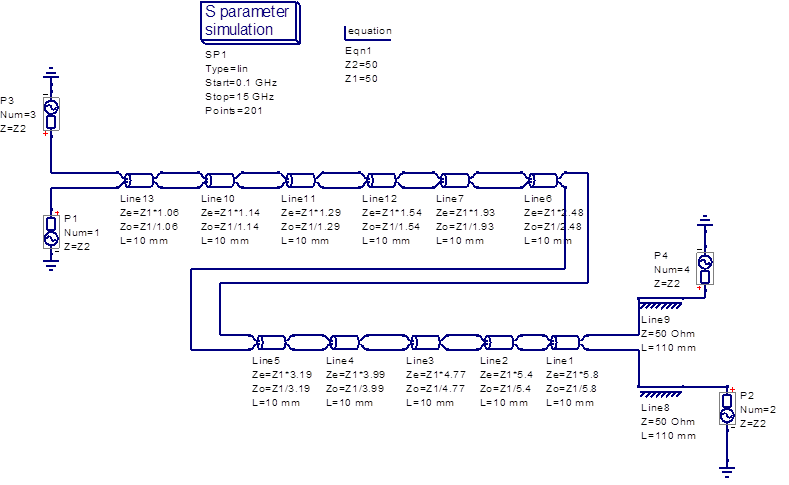The performance of the coupler is shown below. There is equal power division (3 dB) at the output ports when the Delta (port 1) and Sum (port 4) are fed. The coupler works above 2 GHz (the cutoff frequency of the Klopfenstein taper). The poor performance above 12 GHz is due to the fact that I only discretized the lines into 11 sections. At 15 GHz, the 10 mm transmission lines sections are half-wavelength, and don’t represent a gradual taper. The phase difference of the output ports when the Delta and Sum ports are also shown below. There is roughly 0.25 dB amplitude ripple and 5 degree phase ripple across the operating bandwidth in this ideal circuit model example. In practice, 1 dB amplitude ripple and 10 degree phase ripple are more typical for commercial couplers that operate over such a wide bandwidth. Note that the return loss (S11) and isolation (S41) are both exactly 0 in this example since the circuit satisfies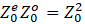at every point.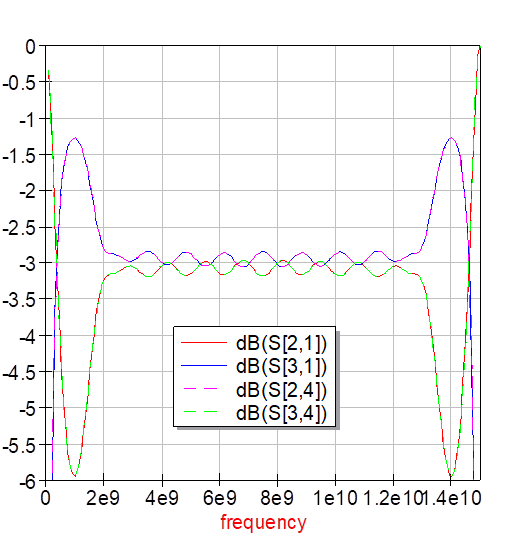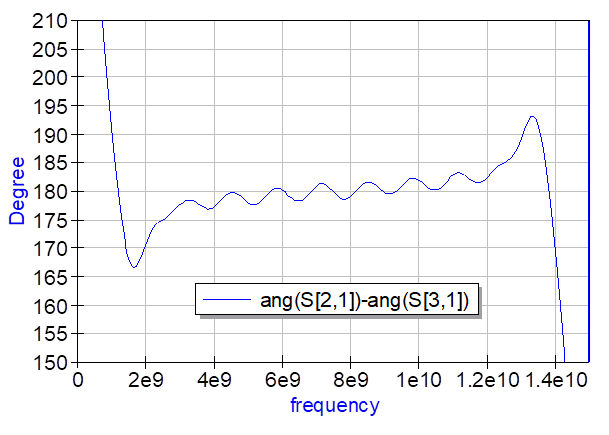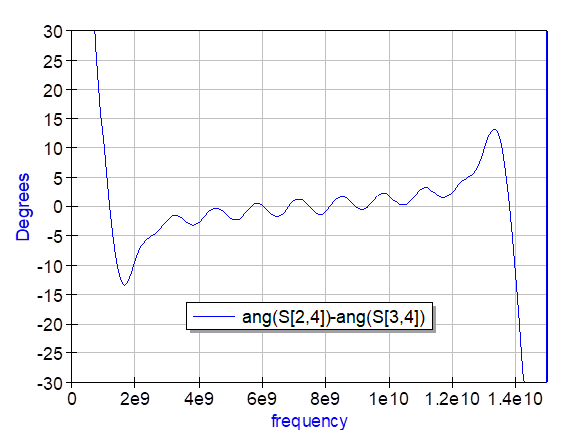While it is possible to physically implement the single stage 3 dB coupler shown above, it can be very challenging to realize a coupling coefficient as high as k=5.8. Therefore, tandem 8.34 dB couplers are often used instead to realize an 3 dB coupler, as shown below. This approach only requires a max coupling coefficient of k=2.23. However, the amplitude ripple is larger, as shown below. Again, I used the Klopfenstein taper calculator to calculate the impedances of the coupled lines.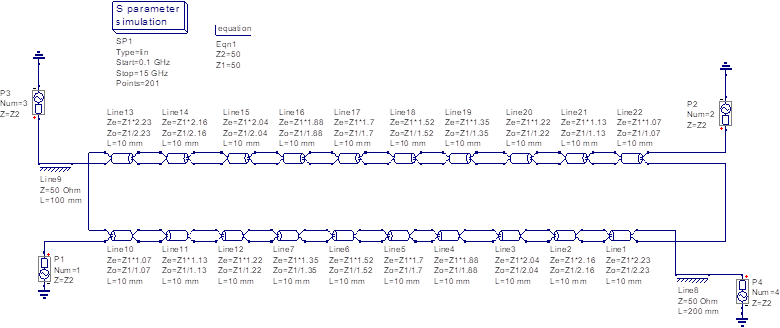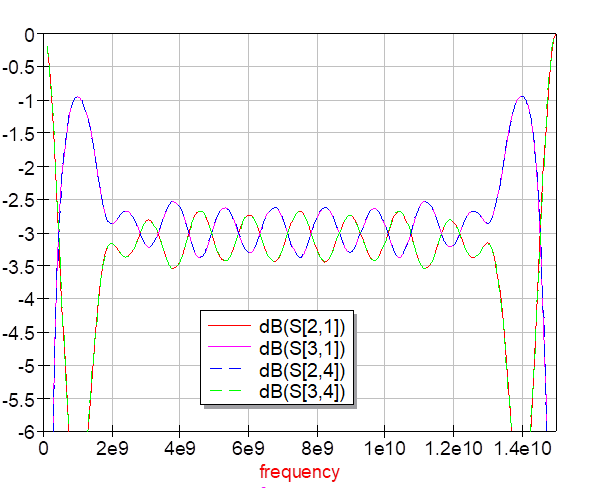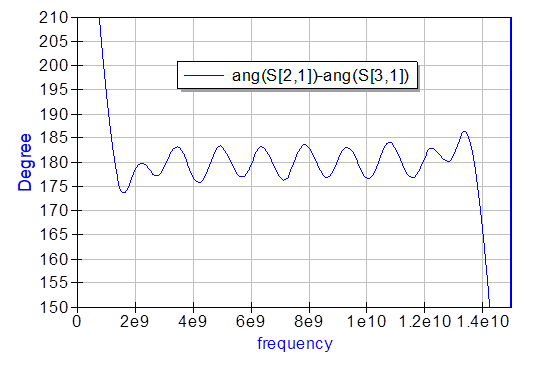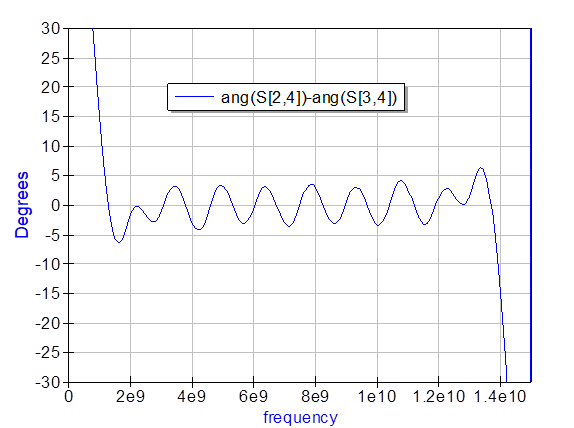Author : Carl Pfeiffer